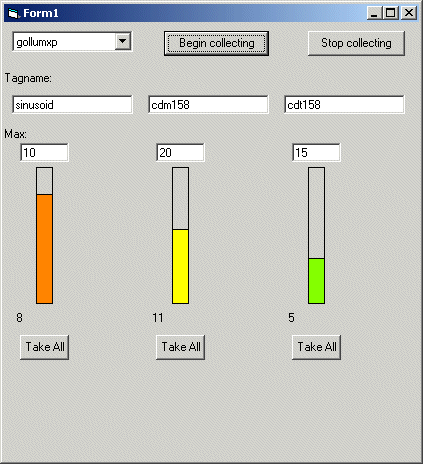# EventPipe ExampleThis example, during the Form_Load, populates the edit boxes with tags whose events will be collected.  A PI-SDK control (server pick list) allows selection of the desired server.  When the "Begin collecting" button is hit, the application connects to the selected server, grabs an EventPipe for each tag and sets the pipe's MaxCount property to the value in the corresponding text box.  A timer is enabled at this point to periodically check the pipes for values.  On each timer event the app gets the count from each event pipe and graphically displays how full the pipe is.  When the "Take All" button is clicked under any tag, EventPipe.TakeAll is called and the value of the most recent event (highest index) is dispayed below the button. with the timestamp shown just below.

Build the EventPipe example as follows:

1. Create a new project and add a reference to the PISDK library.

2. Add a reference to the PI-SDK Control under the Project | Components menu.

3. Add the visual elements as shown in the picture. Name the objects as follows

Object Name
Begin Collecting button btnBegin
Stop Collecting button btnStop
Tagname edit boxes txtTagName1 control array
Max edit boxes txtMax1 control array
Rectangles for % full display ShapeFill1control array
Take All buttons btnTakeAll1 control array
Value display labels lblValue1 control array
Timestamp display labels lblTime1 control array
Timer Timer1

4. Cut and paste the code below into the project.

```Dim srv As Server
Dim evp1(3) As EventPipe
Private Sub btnBegin_Click()
Set srv = PISrvPickList1.SelectedServer
If Not srv.Connected Then
Dim cnxn As New Connections
End If
MonitorPipes
End Sub

Private Sub MonitorPipes()
On Error GoTo eh
Dim i As Integer
Dim pt As PIPoint
For i = 0 To 2
Set pt = srv.PIPoints(txtTagName1(i).Text)
Set evp1(i) = pt.Data.EventPipe
evp1(i).maxCount = CInt(txtMax1(i).Text)
Next i
Timer1.Enabled = True
Timer1.Interval = 1000

Exit Sub
eh:
MsgBox Err.Description
End Sub

Private Sub btnStop_Click()
Dim i As Integer
Timer1.Enabled = False
For i = 0 To 2
Set evp1(i) = Nothing
Next i
ClearDisplay
End Sub

Private Sub btnTakeAll1_Click(Index As Integer)
Dim evobjs1() As Object
On Error GoTo eh
If evp1(Index).Count = 0 Then
Exit Sub
End If
Dim endIndex As Long
endIndex = evp1(Index).Count
ReDim evobjs1(1 To evp1(Index).Count)
Dim vtEvents As Variant
vtEvents = evp1(Index).TakeAll
evobjs1 = vtEvents
Dim pievobj As PIEventObject
Set pievobj = evobjs1(endIndex - 1)
Dim pv As PointValue
Set pv = pievobj.EventData
lblValue1(Index).Caption = CStr(pv.PIValue.Value)
lblTime1(Index).Caption = CStr(pv.PIValue.TimeStamp.LocalDate)
Exit Sub
eh:
MsgBox Err.Description
End Sub

Private Sub Timer1_Timer()
' update bar display
Dim maxCount As Single
Dim currentCount As Single
Dim dblBarHeight As Single
Dim dblPercentFull As Single
Dim arrRGB(5) As Long
arrRGB(0) = RGB(0, 255, 0)
arrRGB(1) = RGB(128, 255, 0)
arrRGB(2) = RGB(255, 255, 0)
arrRGB(3) = RGB(255, 128, 0)
arrRGB(4) = RGB(255, 0, 0)

For i = 0 To 2
maxCount = evp1(i).maxCount
currentCount = evp1(i).Count
dblPercentFull = currentCount / maxCount
If dblPercentFull <= 0.2 Then
colorIndex = 0
Else
If dblPercentFull <= 0.4 Then
colorIndex = 1
Else
If dblPercentFull <= 0.6 Then
colorIndex = 2
Else
If dblPercentFull <= 0.8 Then
colorIndex = 3
Else
colorIndex = 4
End If
End If
End If
End If
ShapeFill1(i).FillColor = arrRGB(colorIndex)
dblBarHeight = dblPercentFull * Shape1(i).Height
ShapeFill1(i).Left = Shape1(i).Left
ShapeFill1(i).Width = Shape1(i).Width
ShapeFill1(i).Height = dblBarHeight
ShapeFill1(i).Top = Shape1(i).Top + (Shape1(i).Height - ShapeFill1(i).Height)
lblCurrent1(i).Caption = CStr(evp1(i).Count)
Next i
End Sub
ClearDisplay
txtTagName1(0) = "sinusoid"
txtTagName1(1) = "cdm158"
txtTagName1(2) = "cdt158"
txtMax1(0) = "10"
txtMax1(1) = "20"
txtMax1(2) = "15"
End Sub
Private Sub ClearDisplay()
For i = 0 To 2
ShapeFill1(i).FillStyle = vbFSSolid
ShapeFill1(i).FillColor = RGB(128, 128, 128)
ShapeFill1(i).Left = 0
ShapeFill1(i).Top = 0
ShapeFill1(i).Height = 0
ShapeFill1(i).Width = 0
lblValue1(i).Caption = ""
lblTime1(i).Caption = ""
Next i

End Sub

```
Enabling Operational Intelligence